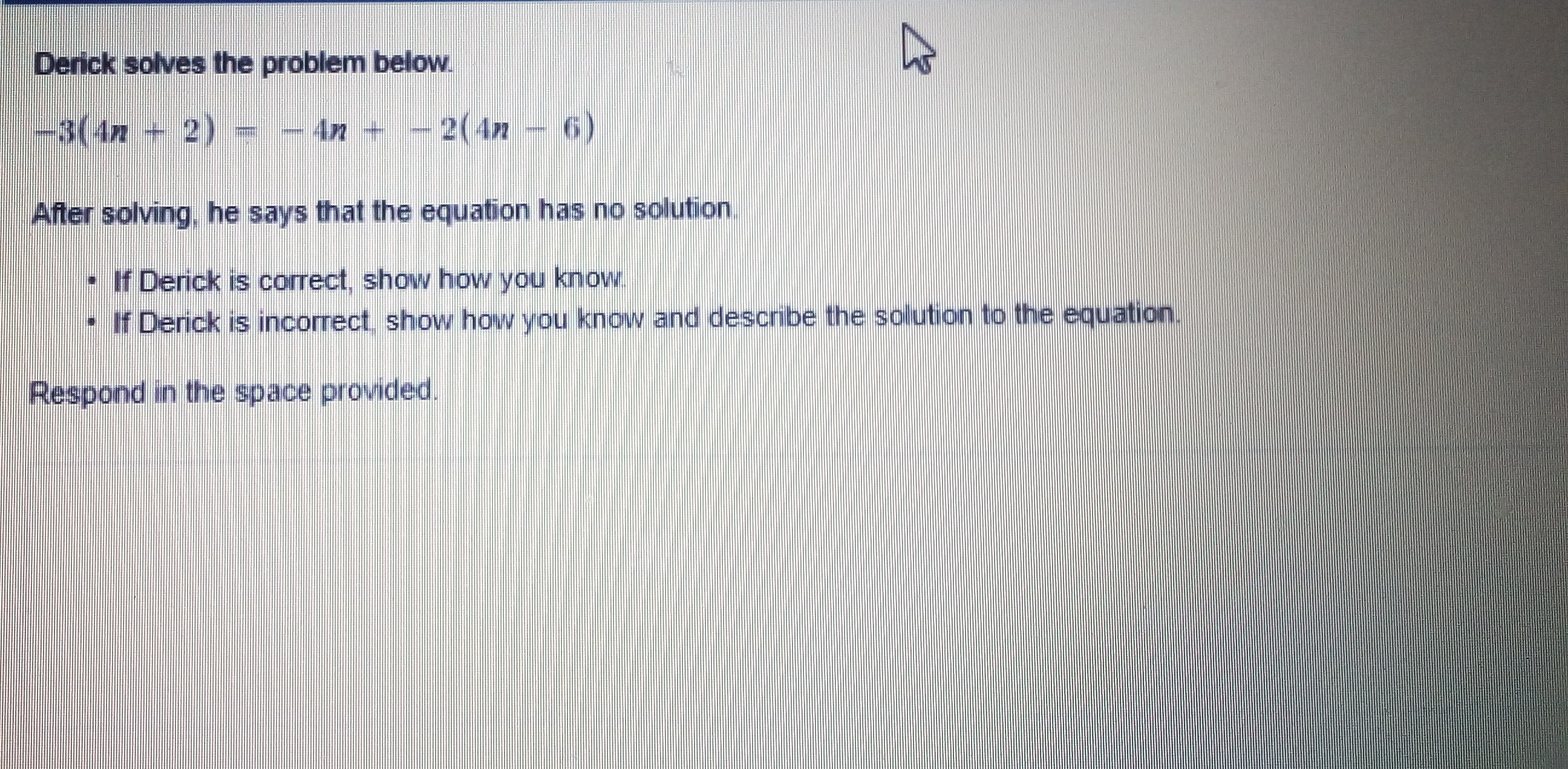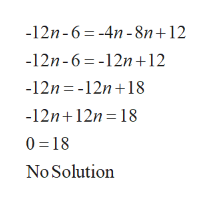# Derick solves the problem below.-3(4n+ 2)4n + - 2(4n – 6)After solving. he says that the equation has no solution.• If Derick is correct, show how you know.- If Derick is incorrect show how you know and describe the solution to the equation.Respond in the space provided.

Question
11 views

Help with responsehelp_outlineImage TranscriptioncloseDerick solves the problem below. -3(4n+ 2) 4n + - 2(4n – 6) After solving. he says that the equation has no solution. • If Derick is correct, show how you know. - If Derick is incorrect show how you know and describe the solution to the equation. Respond in the space provided. fullscreen
check_circle

star
star
star
star
star
1 Rating
Step 1

Given, the problem solved by Derick is

Step 2

And after solving the equation, he says that the equation has no solution

Step 3

Simplify the equat...help_outlineImage Transcriptionclose-12n-6= -4n - 8n+12 -12n-6=-12n+12 -12n = -12n+18 -12n+12n= 18 0 = 18 No Solution fullscreen

### Want to see the full answer?

See Solution

#### Want to see this answer and more?

Solutions are written by subject experts who are available 24/7. Questions are typically answered within 1 hour.*

See Solution
*Response times may vary by subject and question.
Tagged in

### Numbers